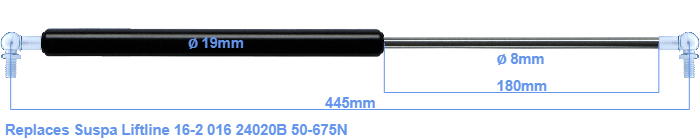# Replacement for Suspa Liftline 16-2 016 24020B 50-675N

\$51.51

Replacement gas spring for the Suspa Liftline 16-2 016 24020B 50-675 Newton. This fits a (possibly already present) ball with a diameter of 10mm. A corresponding ball is included. Brand: Hahn.
 Force Choose an option50 Newton60 Newton80 Newton100 Newton120 Newton140 Newton150 Newton160 Newton180 Newton200 Newton220 Newton240 Newton250 Newton260 Newton280 Newton300 Newton320 Newton340 Newton350 Newton360 Newton380 Newton400 Newton420 Newton440 Newton450 Newton460 Newton480 Newton500 Newton520 Newton540 Newton550 Newton560 Newton580 Newton600 Newton620 Newton640 Newton650 Newton660 Newton675 NewtonClearThis gas spring has a cylinder diameter of 19 mm. The rod has a diameter of 8 mm. The stroke (the retracting part) has a length of 180 mm. In total, the length is 445 millimeter. This is the distance between the centers of the mounting parts. Without the mounting parts this gas spring is 405 mm long (thread to thread). This replacement gas spring (16-2 016 24020B) has a force of 50-675 Newton (N). This gas spring can replace a Suspa Liftline gas spring, but is not an orignal Suspa Liftline one. This a gas spring with the brand Stabilus Industry Line. The force (50-675 N) and dimensions are identical to the original.
Category: